Ch 27 - Keynesian Macro Model (I)

Sunday, February 05, 2012

1:00 AM

Keynesian Model assumptions:

• Prices stay constant
• No supply constraints

Expenditure and GDP

• Increase in read GDP increase aggregate expenditure
• Increase in aggregate expenditure increases real GDP

 Induced Expenditure Consumption M (imports) Autonomous Expenditure Investment Government Expenditure X (Exports)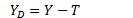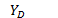Disposable income (spent on consumption or savings) Y Aggregate income T Net taxes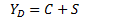C Consumption S Savings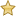Consumption function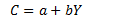C Consumption b (aka MPC) Marginal propensity to consume Y Income (assuming no taxes)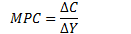MPC Ratio of change in consumption and change disposable income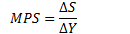MPS Marginal propensity to save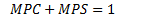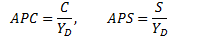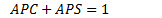APC Average propensity to consume (falls as level of income rises) APS Average propensity to save

Move along the consumption function

• Disposable income

Shifts consumption function:

• Real interest rates (inverse)
• People consume more when borrowing is cheap
• Wealth
• Expected future income (direct)Planned Investment Spending

Determinants of aggregate investment expenditure:

• Real interest rate
• Changes in level of sales

 Real interest rate Opportunity cost of using money for investment in fixed goods Opportunity cost of holding an inventory Affect residential construction Inversely affect Investment expenditure Changes in Sales Directly affect stock of inventories Business confidence Direct affectAggregate Expenditure Function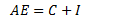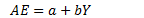a Autonomous expenditure (when disposable income = 0) b MPC (assuming no gov and foreign sector)

 Equilibrium expenditure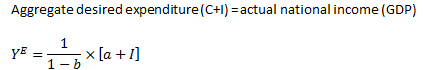Shifts the AE function:

• Autonomous spending
• I, G, X

Changes the slope of AE:

• Change in MPCMultiplier

 Multiplier Amount which a change in autonomous expenditure is magnified to determine the change in equilibrium expenditure and real GDP.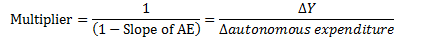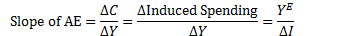Created by Tim Pei with Microsoft OneNote 2010
One place for all your notes and information